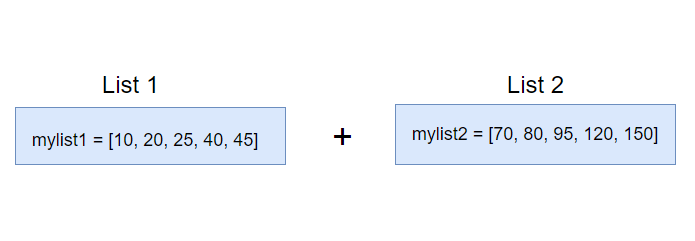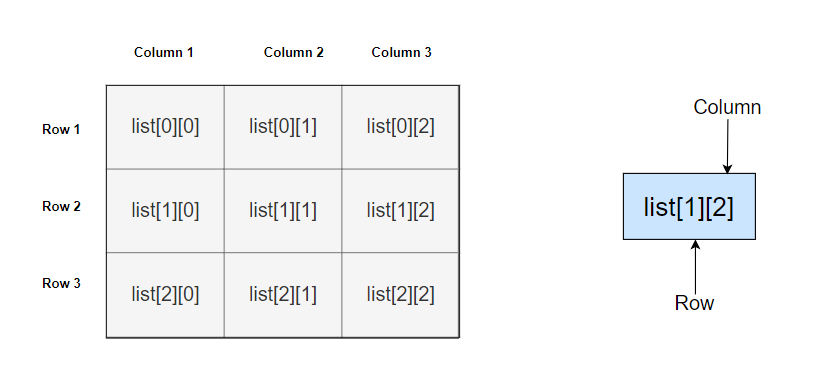## 30 May Python Lists with Examples

Lists in Python are ordered. It is modifiable and changeable, unlike Tuples. Tuples in Python are immutable, whereas Lists are mutable. In other words, the Lists can be modified even after it’s created.

## How to create a Python List

To create a List, you need to set all the items in square brackets []. Each element is separated by commas i.e. comma separated values. Lists offset begins from index 0. Following is an example with 10 integer elements in a List:

Another example showing 5 string elements in a List:

Let us see another list with type float:

List can also have mixed datatype:

Following is an example with output displaying 3 Lists with different datatypes;

The output is as follows:

## Access values from a List

Refer the index number of the specific value you want to access from a List, for example, to access the element at 3rd position, set index 2,

Let us now see an example to access values from a Python List:

The output is as follows:

## How to update list values

Lists are mutable and can be easily updated. Use the slice & assignment operator to set the element’s index on its left and the new value on the right, for example:

Above, we updated the 3rd position (index x2) with the value 50 using slice and assignment operator. Next, let us now see an example wherein we will be updating three different lists in a single Python program:

The output is as follows:

## Remove elements from the List

To remove elements from List, use the del keyword or the remove() method, for example:

Above, deletes the element at index 4.

Let us see an example and remove elements from the List:

The output is as follows:

## Remove element from a specific position (index) in the List

To remove an element from a specific position in List, the del keyword is used. Set the index in the square brackets as in the below example:

The output is as follows:

## Remove a specific element from a Python List

To remove a specific element from a list, set the element to be deleted in the remove() method:

The output is as follows:

## Remove all elements from the List (Delete the entire List)

To remove all elements from List i.e. deleting the entire List is possible with the del keyword. Let us see an example wherein we will delete all the elements from the List:

The output is as follows:

Above, we are getting an error since we are trying to display the List which we deleted using the del keyword.

## Remove multiple elements from a List in Python

To remove/delete multiple elements from a Python List, use the del keyword as in the below example. Set the range of elements to be deleted:

Above, we deleted elements from 2 (including) to 4 (excluding) indexes. Therefore, we deleted two elements i.e. index 2 and index 3.

Let us now see an example to delete multiple elements:

The output is as follows:

## Indexing in Lists

For indexing in Lists, use the index operator []. The indexing in Lists begins from 0. The last item is tuple length – 1. Set the index in square brackets and fetch a specific element at the same index. With that, if you want to count from the right, use negative indexing. The slice operator can also be used to fetch a specific set of sub-elements. This is achieved with the colon operator and allows to fetch multiple items. Let us see them one by one.

Fetch a specific element with indexing in Lists

To fetch a specific element, just set the index in a square bracket i.e. refer to the index number:

Above, we fetched elements using the index numbers. Let us see an example and fetch a specific element with indexing in Lists:

The output is as follows:

## Negative Indexing in Lists

Left to right traversal of List elements are possible with negative indexing. For example, index -1 is the last item. Let us see an example of negative indexing in List:

The output is as follows:

## Slice Lists

Slice Lists in Python using the slice operator (:). The slice operator is used to fetch a specific set of sub-elements i.e. slicing. Let us see an example:

The output is as follows:

In the above example, [2:4] means the search starts from index 2 (including) and ends at index 4 (excluding). In the same way, [2:] means the search starts from 2nd to end.

## Range of indexes

By specifying the start and end range, we can specify the range of indexes in Python. Let us see an example:

Let us now see an example:

The output is as follows:

In the above example, [1:2] means the search starts from index 1 (including) and ends at index 2 (excluding). In the same way, [3:5] means the search starts from 2 (including) and ends at index 5 (excluding):

## Iterate through the List

The for loop is used to iterate through a List in Python as in the below example:

The output is as follows:

## Add elements to the List

To add elements to the List, use the append() method as in the below example:

The output is as follows:

## Add element at a specific index in List

The insert() method is used to add element at a specific index in List as in the below example:

Above, the first parameter in the insert() method is the index and the second parameter is the element to be inserted.

Finally, let us see a complete example:

The output is as follows:

## Check if a specific item exists in a List

To check if a specific element exists in a List, the in keyword is used:

The output is as follows:

## Extend the List by adding another List to the end

The extend() method is used to extend the list by adding another List to the end. Let us see an example wherein we are extending a List:

The output is as follows:

## Get the length of the List

To get the length of the List, the len() method is used in Python. Let us see an example to get the total length of the List:

The output is as follows:

## Get maximum value from the List

To get the maximum value from the List, the max() method is to be used. Let us see an example:

The output is as follows:

## Get minimum value from the List

To get the minimum value from the List, the min() method is to be used. Let us see an example:

The output is as follows:

## Make a copy of the List

To make a copy of an already created list, the copy() method is used. Let us see an example:

The output is as follows:

## Join Two Lists in Python

The + operator is used to join two lists in Python as in the below illustration:Let us now see an example wherein we will join two lists:

The output is as follows:

## Concatenate Two Lists in Python

The + operator is used to concatenate two lists in Python as in the below example:

The output is as follows:

## Sort List in Python

The sort() method is used in Python to sort List as in the below example:

The output is as follows:

## Reverse the order of the List

To reverse the order of elements in a List, the reverse() method is to be used. Let us now see an example:

The output is as follows:

## Multi-Dimensional Python List

Lists within Lists are known as Multi-Dimensional Lists in Python. Let us first see how to create a Multi-Dimensional Python List:

Above, we created 4 integer lists in a List i.e. Multi-dimensional List. To access a list in a multi-dimensional list, use square brackets, like Multi-Dimensional Arrays with:

We are accessing the first and second elements in the 1st list of our Multi-Dimensional List:

To explain the concept, we have represented Multi-dimensional List graphically below. Similarly, we represent the left index as row number and right index as column number:Finally, let us see an example wherein we will be creating a Multi-Dimensional List and learn to display all the elements or any specific element:

The output is as follows:

## Access elements in a Multi-Dimensional Python List

To access elements in a Multi-Dimensional List, use the number of rows and number of columns. For example, to access the 1st element of the first row set the index of rows and columns i.e. for the 1st element:

For the 2nd element in the first row:

In the same way, for the 1st element in the second row:

Finally, let us see an example to access elements in a Multi-Dimensional List with 4 lists:

The output is as follows:

## Add elements to the end of the Multi-Dimensional List

Adding elements to the end of Multi-Dimensional List is possible with the append() method as in the below example:

The output is as follows:

In this tutorial, we learned what are Lists in Python. Moreover, we worked around creating Python Lists and their operations like adding, deleting, extending, appending, etc. Clearly, it’s quite easy to work on Python Lists. Apart from this, if you are aware of a topic we missed in the List, then kindly mention it in the comment section.

Recommend Posts[email protected]

We work to create programming tutorials for all.